Systems of Linear Equations: Warnings (page 3 of 7)

Sections: Definitions, Solving by graphing, Substitition, Elimination/addition, Gaussian elimination.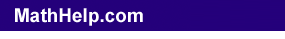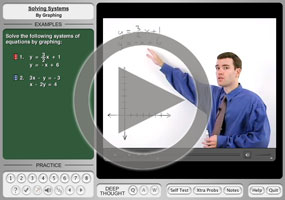Most "solving by graphing" problems work nicely, but sometimes they'll give you an inconsistent system (that is, two parallel lines) or a dependent system (that is, two forms of the same line equation). This is what these cases will look like:

• Solve the following system by graphing.
• y = 36 – 9x
3
x + y/3 = 12

As usual, I'll first solve the equations for "y =". The first equation, in this case, is already solved, so now I'll solve the second one: Copyright © Elizabeth Stapel 2003-2011 All Rights Reserved

3x + y/3 = 12
9x + y = 36
y = 36 – 9x

With both equations solved for "y =", I can see that these two equations are really both the same line! So the algebra tells me that this is a dependent system, and the solution is the whole line. Of course, this is a "solving by graphing" problem, so I still have to do the graph, but I already know the answer.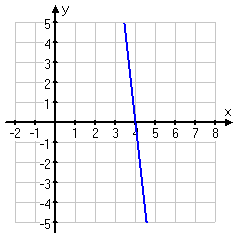solution:  y = 36 – 9x

The solution to this system is the whole line, so, in my classes, you could give the answer as being "y = 36 – 9x". However, most books do something like this: You are looking for an x,y-solution, and, in this case, x = x and y = 36 – 9x, so the solution "point" is of the form (x, 36 – 9x). But then the book does this weird thing with "a" (or "t" or "s" or some other variable). Instead of using x, which is a perfectly good variable, they pull out this new variable from behind their left ear and give the solution as being "(a, 36 – 9a)". I have no idea why they do this, but if your book does this, then (Warning!) that is the format that your teacher will want on the test. Make sure you memorize the variable that your particular book uses (which was "a" in this example).

• Solve the following system by graphing.
• 7x + 2y = 16
–21
x – 6y = 24

As usual, I'll first solve each equation for "y =":

7x + 2y = 16
2y = –7x + 16
y = –( 7/2 )x + 8

–21x – 6y = 24
–21x – 24 = 6y
–( 21/6 )x – 4 = y
–( 7/2 )x – 4 = y

These lines have the same slope — namely, m = –7/2  — but different y-intercepts, so they are parallel. Since parallel lines never cross, the algebra tells me that this is an inconsistent system; that is, there is no solution. But this is a "solving by graphing" problem, so I still have to draw the picture.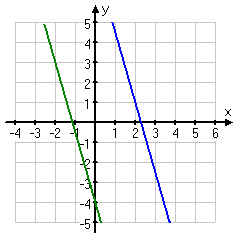solution: no solution (inconsistent system)

Warning: When the algebra tells you that you have two parallel lines, for heaven's sake, draw the lines on your graph so they look parallel!

Note: The solution to a dependent system, being all the points along the line, contains infinitely-many points. But don't make the mistake of thinking that "infinitely-many" means "all". Any point off the line is not a solution; only the infinity of points actually on the line will solve the dependent system.

 Also note: The pictures on the first page of this lesson are very useful for explaining "what's going on" with linear systems, but pictures are not terribly useful for finding actual solutions to systems. For instance, in the picture at right, is the solution point at (–3, 2), or at (–3.15, 1.97)?    You can't tell!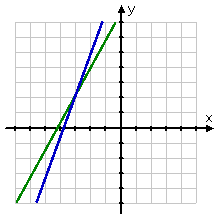Or, in the picture at right, are the lines really parallel, so there's no solution? Or are you just looking at an un-useful portion of the graph?       You can't tell!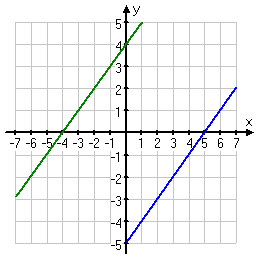In this case, zooming out shows that the lines in the previous picture do indeed cross, at the point (450, 449.5). But this was not at all apparent in the "standard" viewing window shown above.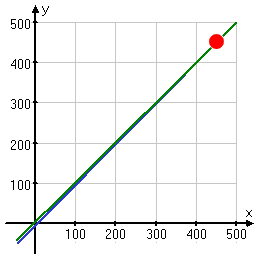So you can see that the pictures can be useful, especially for the concepts, but you should take "solving by graphing" with a grain of salt, and should keep in mind that the algebraic techniques (rather than mere pictures) are the tools you need for solid answers.

Content Continues Below

The above discussion was specific to the two-equation, two-variable case, because you can draw pictures of the two-variable case to illustrate what is going on. But the terminology and basic concepts are the same, no matter how many variables you have. You could have four equations in four variables or twelve equations in twelve variables, and you would still be looking for where the "lines" "intersect" — you just couldn't draw a picture of it.

Formatting note: For reasons which will become apparent when you start working with matrices, equations in systems of equation are generally written with the variables on the left-hand side of the "equals" sign and the numbers on the right-hand side. Sometimes you'll find a question formatted differently, but variables-on-the-left will be the norm.

<< Previous  Top  |  1 | 2 | 3 | 4 | 5 | 6 | 7  |  Return to Index  Next >>

 Cite this article as: Stapel, Elizabeth. "Systems of Linear Equations: Warnings." Purplemath. Available from     https://www.purplemath.com/modules/systlin3.htm. Accessed [Date] [Month] 2016

MathHelp.com Courses

Purplemath:

Study Skills Survey